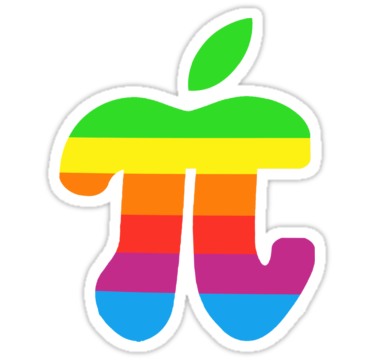Search IntMath
Close

450+ Math Lessons written by Math Professors and Teachers

5 Million+ Students Helped Each Year

1200+ Articles Written by Math Educators and Enthusiasts

Simplifying and Teaching Math for Over 23 Years

# Pi Day today, 3.14

By Murray Bourne, 14 Mar 2012

March 14th is celebrated worldwide as "Pi Day" by math nerds.

I enjoyed this relevant sticker I found recently:Image source: Apple Pi sticker, by Jeff Cheung.

## Pi Day info

Check the Exploratorium's Brief History of Pi

Here's a controversial proposal: Let's Drop Pi

TeachPi.org have a list of possible Pi Day activities you can consider at your school.

Anyone see the Pi Movie, from 1998?

And finally, here's a lot of good info about pi.

Be the first to comment below.

### Comment Preview

HTML: You can use simple tags like <b>, <a href="...">, etc.

To enter math, you can can either:

1. Use simple calculator-like input in the following format (surround your math in backticks, or qq on tablet or phone):
a^2 = sqrt(b^2 + c^2)
(See more on ASCIIMath syntax); or
2. Use simple LaTeX in the following format. Surround your math with $$ and $$.
$$\int g dx = \sqrt{\frac{a}{b}}$$
(This is standard simple LaTeX.)

NOTE: You can mix both types of math entry in your comment.